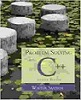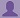SHARE
HELP

To start with, Sr2Jr’s first step is to reduce the expenses related to education. To achieve this goal Sr2Jr organized the textbook’s question and answers. Sr2Jr is community based and need your support to fill the question and answers. The question and answers posted will be available free of cost to all.# Problem Solving With C++

Authors:
Walter Savitch ,julia Lobur
Exercise:
Programming Projects
Chapter:
Introduction To Computers And C++ Programming
Edition:
7
ISBN:
9780321531346
Question:
3
 Previous Next

###Question

Further modify the C++ program that you already have modified in Programming Project 2. Change the multiplication sign * in your C++ program to a division sign /. Recompile the changed program. Run the program. Enter a zero input for “number of peas in a pod.” Notice the run time error message due to division by zero.

###Answer

﻿Modified program code:

``````
#include<iostream>
using namespace std;

//main function
int main( )
{
//Variable declaration
int number_of_pods, peas_per_pod, total_peas;
//Prompt the message, Hello
cout << "Hello\n";

//Prompt the user to enter input
cout << "Press return after entering a number.";
cout << "Enter the number of pods:";
cin >> number_of_pods;
cout << "Enter the number of peas in a pod:";
cin >> peas_per_pod;
//Calculation part by using division symbole
total_peas = number_of_pods / peas_per_pod; //This line
//Display output
cout << "If you have ";
cout << number_of_pods;
cout << " pea pods ";
cout << "and ";
cout << peas_per_pod;
cout << " peas in each pod, then ";
cout << "you have ";
cout << total_peas;
cout << " peas in all the pods. ";

//Prompt the message, Good-bye
cout << "Good-bye\n";

return 0;
}
``````

Output:

``````Hello
Press return after entering a number.Enter the number of pods:200

Enter the number of peas in a pod:10
If you have 200 pea pods and 10 peas in each pod, then you have 20 peas in all t
he pods. Good-bye``````

### Discussions

Post the discussion to improve the above solution.